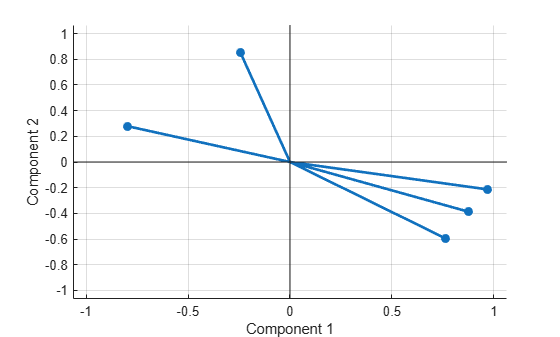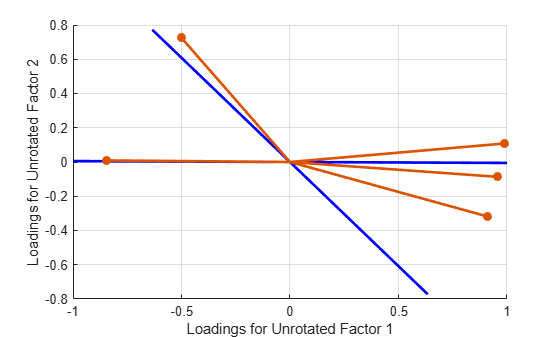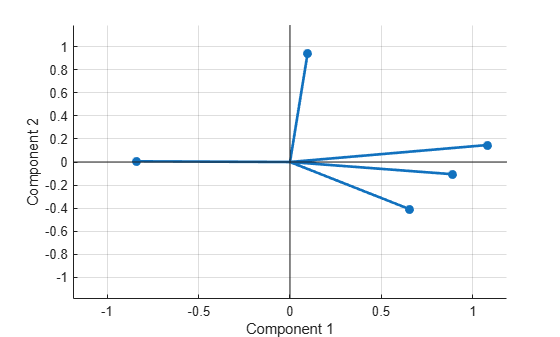# factoran

Factor analysis

## Syntax

``lambda = factoran(X,m)``
``[lambda,psi] = factoran(X,m)``
``[lambda,psi,T] = factoran(X,m)``
``[lambda,psi,T,stats] = factoran(X,m)``
``[lambda,psi,T,stats,F] = factoran(X,m)``
``___ = factoran(X,m,Name,Value)``

## Description

`factoran` computes the maximum likelihood estimate (MLE) of the factor loadings matrix Λ in the factor analysis model

`$x=\mu +\Lambda f+e$`

where x is a vector of observed variables, μ is a constant vector of means, Λ is a constant `d`-by-`m` matrix of factor loadings, f is a vector of independent, standardized common factors, and e is a vector of independent specific factors. x, μ, and e each has length `d`. f has length `m`.

Alternatively, the factor analysis model can be specified as

`$\mathrm{cov}\left(x\right)=\Lambda {\Lambda }^{T}+\Psi$`

where $\Psi =\mathrm{cov}\left(e\right)$ is a `d`-by-`d` diagonal matrix of specific variances.

For the uses of `factoran` and its relation to `pca`, see Perform Factor Analysis on Exam Grades.

example

````lambda = factoran(X,m)` returns the factor loadings matrix `lambda` for the data matrix `X` with `m` common factors.```

example

````[lambda,psi] = factoran(X,m)` also returns maximum likelihood estimates of the specific variances.```

example

````[lambda,psi,T] = factoran(X,m)` also returns the `m`-by-`m` factor loadings rotation matrix `T`.```

example

````[lambda,psi,T,stats] = factoran(X,m)` also returns the structure `stats` containing information relating to the null hypothesis H0 that the number of common factors is `m`.```

example

````[lambda,psi,T,stats,F] = factoran(X,m)` also returns predictions of the common factors (factor scores).```

example

````___ = factoran(X,m,Name,Value)` modifies the model fit and outputs using one or more name-value pair arguments, for any output arguments in the previous syntaxes. For example, you can specify that the `X` data is a covariance matrix.```

## Examples

collapse all

Create some pseudorandom raw data.

```rng default % For reproducibility n = 100; X1 = 5 + 3*rand(n,1); % Factor 1 X2 = 20 - 5*rand(n,1); % Factor 2```

Create six data vectors from the raw data, and add random noise.

```Y1 = 2*X1 + 3*X2 + randn(n,1); Y2 = 4*X1 + X2 + 2*randn(n,1); Y3 = X1 - X2 + 3*randn(n,1); Y4 = -2*X1 + 4*X2 + 4*randn(n,1); Y5 = 3*(X1 + X2) + 5*randn(n,1); Y6 = X1 - X2/2 + 6*randn(n,1);```

Create a data matrix from the data vectors.

`X = [Y1,Y2,Y3,Y4,Y5,Y6];`

Extract the two factors from the noisy data matrix `X` using `factoran`. Display the outputs.

```m = 2; [lambda,psi,T,stats,F] = factoran(X,m); disp(lambda)```
``` 0.8666 0.4828 0.8688 -0.0998 -0.0131 -0.5412 0.2150 0.8458 0.7040 0.2678 -0.0806 -0.2883 ```
`disp(psi)`
``` 0.0159 0.2352 0.7070 0.2385 0.4327 0.9104 ```
`disp(T)`
``` 0.8728 0.4880 0.4880 -0.8728 ```
`disp(stats)`
``` loglike: -0.0531 dfe: 4 chisq: 5.0335 p: 0.2839 ```
`disp(F(1:10,:))`
``` 1.8845 -0.6568 -0.1714 -0.8113 -1.0534 2.0743 1.0390 -1.1784 0.4309 0.9907 -1.1823 0.6570 -0.2129 1.1898 -0.0844 -0.7421 0.5854 -1.1379 0.8279 -1.9624 ```

View the correlation matrix of the data.

`corrX = corr(X)`
```corrX = 6×6 1.0000 0.7047 -0.2710 0.5947 0.7391 -0.2126 0.7047 1.0000 0.0203 0.1032 0.5876 0.0289 -0.2710 0.0203 1.0000 -0.4793 -0.1495 0.1450 0.5947 0.1032 -0.4793 1.0000 0.3752 -0.2134 0.7391 0.5876 -0.1495 0.3752 1.0000 -0.2030 -0.2126 0.0289 0.1450 -0.2134 -0.2030 1.0000 ```

Compare `corrX` to its corresponding values returned by `factoran`, `lambda*lambda' + diag(psi)`.

`C0 = lambda*lambda' + diag(psi)`
```C0 = 6×6 1.0000 0.7047 -0.2726 0.5946 0.7394 -0.2091 0.7047 1.0000 0.0426 0.1023 0.5849 -0.0413 -0.2726 0.0426 1.0000 -0.4605 -0.1542 0.1571 0.5946 0.1023 -0.4605 1.0000 0.3779 -0.2611 0.7394 0.5849 -0.1542 0.3779 1.0000 -0.1340 -0.2091 -0.0413 0.1571 -0.2611 -0.1340 1.0000 ```

`factoran` obtains `lambda` and `psi` that correspond closely to the correlation matrix of the original data.

View the results without using rotation.

```[lambda,psi,T,stats,F] = factoran(X,m,'Rotate','none'); disp(lambda)```
``` 0.9920 0.0015 0.7096 0.5111 -0.2755 0.4659 0.6004 -0.6333 0.7452 0.1098 -0.2111 0.2123 ```
`disp(psi)`
``` 0.0159 0.2352 0.7070 0.2385 0.4327 0.9104 ```
`disp(T)`
``` 1 0 0 1 ```
`disp(stats)`
``` loglike: -0.0531 dfe: 4 chisq: 5.0335 p: 0.2839 ```
`disp(F(1:10,:))`
``` 1.3243 1.4929 -0.5456 0.6245 0.0928 -2.3246 0.3318 1.5356 0.8596 -0.6544 -0.7114 -1.1504 0.3947 -1.1424 -0.4358 0.6065 -0.0444 1.2789 -0.2350 2.1169 ```

Compute the factors using only the covariance matrix of `X`.

```X2 = cov(X); [lambda2,psi2,T2,stats2] = factoran(X2,m,'Xtype','covariance','Nobs',n)```
```lambda2 = 6×2 0.8666 0.4828 0.8688 -0.0998 -0.0131 -0.5412 0.2150 0.8458 0.7040 0.2678 -0.0806 -0.2883 ```
```psi2 = 6×1 0.0159 0.2352 0.7070 0.2385 0.4327 0.9104 ```
```T2 = 2×2 0.8728 0.4880 0.4880 -0.8728 ```
```stats2 = struct with fields: loglike: -0.0531 dfe: 4 chisq: 5.0335 p: 0.2839 ```

The results are the same as with the raw data, except `factoran` cannot compute the factor scores matrix `F` for covariance data.

`load carbig`

Define the variable matrix.

```X = [Acceleration Displacement Horsepower MPG Weight]; X = X(all(~isnan(X),2),:);```

Estimate the factor loadings using a minimum mean squared error prediction for a factor analysis with two common factors.

```[Lambda,Psi,T,stats,F] = factoran(X,2,'Scores','regression'); inv(T'*T); % Estimated correlation matrix of F, == eye(2) Lambda*Lambda' + diag(Psi); % Estimated correlation matrix Lambda*inv(T); % Unrotate the loadings F*T'; % Unrotate the factor scores```

Create a biplot of two factors.

`biplot(Lambda,'LineWidth',2,'MarkerSize',20)``[Lambda,Psi,T] = factoran(cov(X),2,'Xtype','covariance')`
```Lambda = 5×2 -0.2432 -0.8500 0.8773 0.3871 0.7618 0.5930 -0.7978 -0.2786 0.9692 0.2129 ```
```Psi = 5×1 0.2184 0.0804 0.0680 0.2859 0.0152 ```
```T = 2×2 0.9476 0.3195 0.3195 -0.9476 ```

(You could instead use `corrcoef(X)` instead of `cov(X)` to create the data for `factoran`.) Although the estimates are the same, the use of a covariance matrix rather than raw data prevents you from requesting the scores or significance level.

Use promax rotation.

```[Lambda,Psi,T,stats,F] = factoran(X,2,'Rotate','promax','power',4); inv(T'*T) % Estimated correlation of F, no longer eye(2)```
```ans = 2×2 1.0000 -0.6391 -0.6391 1.0000 ```
`Lambda*inv(T'*T)*Lambda'+diag(Psi) % Estimated correlation of X`
```ans = 5×5 1.0000 -0.5424 -0.6893 0.4309 -0.4167 -0.5424 1.0000 0.8979 -0.8078 0.9328 -0.6893 0.8979 1.0000 -0.7730 0.8647 0.4309 -0.8078 -0.7730 1.0000 -0.8326 -0.4167 0.9328 0.8647 -0.8326 1.0000 ```

Plot the unrotated variables with oblique axes superimposed.

```invT = inv(T); Lambda0 = Lambda*invT; figure() line([-invT(1,1) invT(1,1) NaN -invT(2,1) invT(2,1)], ... [-invT(1,2) invT(1,2) NaN -invT(2,2) invT(2,2)], ... 'Color','r','LineWidth',2) grid on hold on biplot(Lambda0,'LineWidth',2,'MarkerSize',20) xlabel('Loadings for unrotated Factor 1') ylabel('Loadings for unrotated Factor 2')```Plot the rotated variables against the oblique axes.

```figure() biplot(Lambda,'LineWidth',2,'MarkerSize',20)```## Input Arguments

collapse all

Data, specified as an `n`-by-`d` matrix, where each row is an observation of `d` variables.

Data Types: `double`

Number of common factors, specified as a positive integer.

Example: 3

Data Types: `double`

### Name-Value Arguments

Specify optional comma-separated pairs of `Name,Value` arguments. `Name` is the argument name and `Value` is the corresponding value. `Name` must appear inside quotes. You can specify several name and value pair arguments in any order as `Name1,Value1,...,NameN,ValueN`.

Example: `lambda = factoran(X,m,'Start',10,'Scores','Thomson')` specifies to use a starting point for specific variances of 10 and the Thomson method for predicting factor scores.

Input data type of `X`, specified as the comma-separated pair consisting of `'Xtype'` and one of the following:

• `'data'``X` is raw data.

• `'covariance'``X` is a positive definite covariance or correlation matrix.

Example: `'Xtype','covariance'`

Data Types: `char` | `string`

Method for predicting factor scores, specified as the comma-separated pair consisting of `'Scores'` and one of the following:

• `'wls'` or the equivalent `'Bartlett'` — Weighted least-squares estimate treating `F` as fixed

• `'regression'` or the equivalent `'Thomson'` — Minimum mean squared error prediction that is equivalent to a ridge regression

Example: `'Scores','regression'`

Data Types: `char` | `string`

Starting point for the specific variances `psi` in the maximum likelihood optimization, specified as the comma-separated pair consisting of `'Start'` and one of the following:

• `'Rsquared'` — Chooses the starting vector as a scale factor times `diag(inv(corrcoef(X)))` (default). For examples, see Jöreskog .

• `'random'` — Chooses `d` uniformly distributed values on the interval [0,1].

• Positive integer — Performs the given number of maximum likelihood fits, each initialized in the same way as `'random'`. `factoran` returns the fit with the highest likelihood.

• Matrix with `d` rows — Performs one maximum likelihood fit for each column of the specified matrix. `factoran` initializes the `i`th optimization with the values from the `i`th column.

Example: `'Start',5`

Data Types: `double` | `char` | `string`

Method used to rotate factor loadings and scores, specified as the comma-separated pair consisting of `'Rotate'` and one of the values in the following table. You can control the rotation by specifying additional name-value pair arguments of the `rotatefactors` function, as described in the table. For details, see `rotatefactors`.

ValueDescription

`'none'`

Performs no rotation

`'equamax'`

Special case of the `'orthomax'` rotation. Use the `'normalize'`, `'reltol'`, and `'maxit'` arguments to control the details of the rotation.

`'orthomax'`

Orthogonal rotation that maximizes a criterion based on the variance of the loadings. Use the `'coeff'`, `'normalize'`, `'reltol'`, and `'maxit'` arguments to control the details of the rotation.

`'parsimax'`

Special case of the orthomax rotation. Use the `'normalize'`, `'reltol'`, and `'maxit'` arguments to control the details of the rotation.

`'pattern'`

Performs either an oblique rotation (the default) or an orthogonal rotation to best match a specified pattern matrix. Use the `'type'` argument to choose the type of rotation. Use the `'target'` argument to specify the pattern matrix.

`'procrustes'`

Performs either an oblique rotation (the default) or an orthogonal rotation to best match a specified target matrix in the least-squares sense. Use the `'type'` argument to choose the type of rotation. Use the `'target'` argument to specify the target matrix.

`'promax'`

Performs an oblique procrustes rotation to a target matrix determined by `factoran` as a function of an orthomax solution. Use the `'power'` argument to specify the exponent for creating the target matrix. Because `'promax'` uses `'orthomax'` internally, you can also specify the arguments that apply to `'orthomax'`.

`'quartimax'`

Special case of the `'orthomax'` rotation. Use the `'normalize'`, `'reltol'`, and `'maxit'` arguments to control the details of the rotation.

`'varimax'`

Special case of the `'orthomax'` rotation (default). Use the `'normalize'`, `'reltol'`, and `'maxit'` arguments to control the details of the rotation.

function handle

Function handle to a rotation function of the form

`[B,T] = myrotation(A,...)`

where `A` is a `d`-by-`m` matrix of unrotated factor loadings, `B` is a `d`-by-`m` matrix of rotated loadings, and `T` is the corresponding `m`-by-`m` rotation matrix.

Use the `factoran` argument `'UserArgs'` to pass additional arguments to this rotation function. See User-Defined Rotation Function.

Example: ```[lambda,psi,T] = factoran(X,m,'Rotate','promax','power',5,'maxit',100)```

Data Types: `char` | `string` | `function_handle`

Lower bound for the `psi` argument during maximum likelihood optimization, specified as the comma-separated pair consisting of `'Delta'` and a scalar value between 0 and 1 (0 < `Delta` < 1).

Example: `0.02`

Data Types: `double`

Options for the maximum likelihood optimization, specified as the comma-separated pair consisting of '`OptimOpts'` and a structure created by `statset`. You can enter `statset('factoran')` for the list of options, which are also described in the following table.

Field Name (`statset` argument)MeaningValue {default}
`'Display'`

Amount of information displayed by the algorithm

• `{'off'}` — Displays no information

• `'final'` — Displays the final output

• `'iter'` — Displays iterative output to the command window for some functions; otherwise displays the final output

`MaxFunEvals`

Maximum number of objective function evaluations allowed

Positive integer, `{400}`
`MaxIter`

Maximum number of iterations allowed

Positive integer, `{100}`
`TolFun`

Termination tolerance for the objective function value. The solver stops when successive function values are less than `TolFun` apart.

Positive scalar, `{1e-8}`
`TolX`

Termination tolerance for the parameters. The solver stops when successive parameter values are less than `TolX` apart.

Positive scalar, `{1e-8}`

Example: `statset('Display','iter')`

Data Types: `struct`

Number of observations used to estimate `X`, specified as the comma-separated pair consisting of `'Nobs'` and a positive integer. `Nobs` applies only when `Xtype` is `'covariance'`. Specifying `'Nobs'` enables you to obtain the `stats` output structure fields `chisq` and `p`.

Example: 50

Data Types: `double`

## Output Arguments

collapse all

Factor loadings, returned as a `d`-by-`m` matrix. `d` is the number of columns of the data matrix `X`, and `m` is the second input argument of `factoran`.

The `(i,j)`th element of `lambda` is the coefficient, or loading, of the `j`th factor for the `i`th variable. By default, `factoran` calls the function `rotatefactors` to rotate the estimated factor loadings using the `'varimax'` option. For information about rotation, see Rotation of Factor Loadings and Scores.

Specific variances, returned as a `d`-by-`1` vector. `d` is the number of columns of the data matrix `X`. The entries of `psi` are maximum likelihood estimates.

Factor loadings rotation, returned as an `m`-by-`m` matrix. `m` is the second input argument of `factoran`. For information about rotation, see Rotation of Factor Loadings and Scores.

Information about the common factors, returned as a structure. `stats` contains information relating to the null hypothesis H0 that the number of common factors is `m`.

`stats` contains the following fields.

FieldDescription
`loglike`

Maximized loglikelihood value

`dfe`

Error degrees of freedom = ```((d-m)^2 - (d+m))/2```

`chisq`

Approximate chi-squared statistic for the null hypothesis

`p`

Right-tail significance level for the null hypothesis

`factoran` does not compute the `chisq` and `p` fields unless `dfe` is positive and all the specific variance estimates in `psi` are positive (see Heywood Case). If `X` is a covariance matrix and you want `factoran` to compute the `chisq` and `p` fields, then you must also specify the `'Nobs'` name-value pair argument.

Factor scores, also called predictions of the common factors, returned as an `n`-by-`m` matrix. `n` is the number of rows in the data matrix `X`, and `m` is the second input argument of `factoran`.

Note

If `X` is a covariance matrix (`Xtype` = `'covariance'`), `factoran` cannot compute `F`.

`factoran` rotates `F` using the same criterion as for `lambda`. For information about rotation, see Rotation of Factor Loadings and Scores.

collapse all

### Heywood Case

If elements of `psi` are equal to the value of the `Delta` parameter (that is, they are essentially zero), the fit is known as a Heywood case, and interpretation of the resulting estimates is problematic. In particular, there can be multiple local maxima of the likelihood, each with different estimates of the loadings and the specific variances. Heywood cases can indicate overfitting (`m` is too large), but can also be the result of underfitting.

Unless you explicitly specify no rotation using the `'Rotate'` name-value pair argument, `factoran` rotates the estimated factor loadings `lambda` and the factor scores `F`. The output matrix `T` is used to rotate the loadings, that is, `lambda = lambda0*T`, where `lambda0` is the initial (unrotated) MLE of the loadings. `T` is an orthogonal matrix for orthogonal rotations, and the identity matrix for no rotation. The inverse of `T` is known as the primary axis rotation matrix, whereas `T` itself is related to the reference axis rotation matrix. For orthogonal rotations, the two are identical.

`factoran` computes factor scores that have been rotated by `inv(T')`, that is, `F = F0 * inv(T')`, where `F0` contains the unrotated predictions. The estimated covariance of `F` is `inv(T'*T)`, which is the identity matrix for orthogonal or no rotation. Rotation of factor loadings and scores is an attempt to create a structure that is easier to interpret in the loadings matrix after maximum likelihood estimation.

### User-Defined Rotation Function

The syntax for passing additional arguments to a user-defined rotation function is:

```[Lambda,Psi,T] = ... factoran(X,2,'Rotate',@myrotation,'UserArgs',1,'two'); ```

 Harman, Harry Horace. Modern Factor Analysis. 3rd Ed. Chicago: University of Chicago Press, 1976.

 Jöreskog, K. G. “Some Contributions to Maximum Likelihood Factor Analysis.” Psychometrika 32, no. 4 (December 1967): 443–82. https://doi.org/10.1007/BF02289658

 Lawley, D. N., and A. E. Maxwell. Factor Analysis as a Statistical Method. 2nd Ed. New York: American Elsevier Publishing Co., 1971.